Posted on

## Panorama – Image registration

The main objective of this project is to create panoramic image from sequence of two or more overlapping images using OpenCV. We assume then overlap of two adjacent images is more than 30%, vertical variation of all images is minimal and images are ordered from left to right.

The main idea of this algorithm is based on independent image stitching of two adjacent images and recurrent stitching of results until complete panorama image isnâ€™t created. The code below shows this idea:

```AutomaticPanorama(vector<Mat> results){			//results contains all input images
vector<Mat> partialResults = vector<Mat>();
while (results.size() != 1){
//Stitch all adjacent images and save result as partial result
for (int i = 0; i < results.size() - 1; i++){
Mat panoramaResult = Panorama(results[i], results[i + 1]);
partialResults.push_back(panoramaResult);
}
//results = paritalResults
vector<Mat> temp = results;
results = partialResults;
partialResults = temp;
partialResults.clear();
}
}
```

Function Panorama(results[i], results[i + 1]) is custom implementation of image stitching. This function uses local detector and descriptor SIFT, Brute-force keypoint matcher and perspective transformation to realize image registration and stitching. Individual steps (parts) of function are described below. In this project we also tried to use other detectors like SURF, ORB and descriptors like SURF, ORB, BRISK and FREAK, but SIFT and SURF appears to be the best choices.

## OpenCV functions

SiftFeatureDetector, SiftDescriptorExtractor, BFMatcher, drawMatches, findHomography, perspectiveTransform, warpPerspective, imshow, imwrite

## Panorama

1. Calculate keypoints for left and right image using SIFT feature detector:
```SiftFeatureDetector detector = SiftFeatureDetector();
vector<KeyPoint> keypointsPrev, keypointsNext;
detector.detect(imageNextGray, keypointsNext);
detector.detect(imagePrevGray, keypointsPrev);
```

2. Calculate local descriptor for all keypoints using SIFT descriptor:
```SiftDescriptorExtractor extractor = SiftDescriptorExtractor();
Mat descriptorsPrev, descriptorsNext;
extractor.compute(imageNextGray, keypointsNext, descriptorsNext);
extractor.compute(imagePrevGray, keypointsPrev, descriptorsPrev);
```
3. For keypoint descriptors from left image find corresponding keypoint descriptors in right image using Brute-Force matcher:
```BFMatcher bfMatcher;
bfMatcher.match(descriptorsPrev, descriptorsNext, matches);
```

4. Find only good matches. Good matches are pairs of keypoints which vertical coordinate variation is less than 5%:
```vector<DMatch> goodMatches;
int minDistance = imagePrevGray.rows / 100 * VERTICALVARIATION;
goodMatches = FindGoodMatches(matches, keypointsPrev, keypointsNext, minDistance);
```

5. Find homography matrix for perspective transformation of right image. Use only good keypoints for computing homography matrix:
```Mat homographyMatrix;
homographyMatrix = findHomography(pointsNext, pointsPrev, CV_RANSAC);
```
6. Warp right image using homography matrix from previous step:
```Mat warpImageNextGray;
warpPerspective(imageNextGray, warpImageNextGray, homograpyMatrix, Size(imageNextGray.cols + imagePrevGray.cols, imageNextGray.rows));
```

7. Calculate left image and right (warped) image corners:
```vector<Point2f> cornersPrev, cornersNext;
SetCorners(imagePrevGray, imageNextGray, &cornersPrev, &cornersNext, homograpyMatrix);
```

8. Find overlap coordinates of left and right images:
```int overlapFromX, overlapToX;
if (cornersNext.x < cornersNext.x){
overlapFromX = cornersNext.x;
}
else{
overlapFromX = cornersNext.x;
}
overlapToX = cornersPrev.x;
```
9. Join left and right (warped) image using linear interpolation in overlapped area. Both images contributes with 100% of its pixels outside the overlapping area. Left image contribute with 100% at the beginning of overlapping area and gradually decreases its contribution to 0% at the end. Right image contribute opposite way from 0% at beginning to 100 % at the end:
```Mat result = Mat::zeros(warpImageNextGray.rows, warpImageNextGray.cols, CV_8UC3);
DrawTransparentImage(imagePrevGray, cornersPrev, warpImageNextGray, cornersNext, &result, overlapFromX, overlapToX);
```
10. Crop joined image:
```Rect rectrangle(0, 0, cornersNext.x, result.rows);
result = result(rectrangle);
```

## Limitation

• Sufficient overlap of adjavent images. We assume more than 30%.
• Maximum number of input images is generally less or equals to 5. The deformation of perspective transformation causes failure of algorithm in larger set of input images.
• Input images has to be sorted for example from left to right.
• Panorama of distance objects gives better results than panorama of nearby objects.

## Comparison of different keypoint detectors and descriptors

Detector / Descriptor Number of all matches Number of good matches Result
SIFT / SIFT 3064 976 Successful
SURF / SURF 3217 1309 Successful
ORB / ORB 3000 1113 Successful
SIFT / BRIEF 2827 790 Successful
SIFT / BRISK 1012 128 Failure
SIFT / FREAK 1154 151 Failure
Posted on

## Object removing in image/videoMarek Grznar

## Introduction

In our project we focus on simple object recognition, then tracking this recognized object and finally we try to delete this object from video. By object recognition we used local features-based methods. We compare SIFT and SURF methods for detection and description. By RANSAC algorithm we compute the homography. In case these algorithms successfully find the object we create a mask where recognized object was white area and the rest was black. By object tracking we compared the two approaches. The first approach is based on calculating optical flow using the iterative Lucas-Kanade method with pyramids. The second approach is based on camshift tracking algorithm. For deleting the object from video we focus to using algorithm based on restoring the selected region in an image using the region neighborhood.

Used functions: floodFill, findHomography, match, fillPoly, goodFeaturesToTrack, calcOpticalFlowPyrLK, inpaint, mixChannels, calcHist, CamShift

## Solution

1. Opening video file, retrieve the next frame (picture), converting from color image to grayscale
```cap.open("Video1.mp4");
cap >> frame;
frame.copyTo(image);
cvtColor(image, gray, COLOR_BGR2GRAY);
```
2. Find object in frame (picture)
1. Keypoints detection and description (SIFT/SURF)
```// SiftFeatureDetector detector( minHessian );
SurfFeatureDetector detector( minHessian );

std::vector<KeyPoint> keypoints_object, keypoints_scene;

detector.detect(img_object, keypoints_object);
detector.detect(img_scene, keypoints_scene);

// SiftDescriptorExtractor extractor;
SurfDescriptorExtractor extractor;

Mat descriptors_object, descriptors_scene;

extractor.compute(img_object, keypoints_object, descriptors_object);
extractor.compute(img_scene, keypoints_scene, descriptors_scene);
```
2. Matching keypoints
```FlannBasedMatcher matcher;
std::vector< DMatch > matches;
matcher.match( descriptors_object, descriptors_scene, matches );
```
3. Homography calculating
```Mat H = findHomography( obj, scene, CV_RANSAC );
```
```cv::Mat mask(img_scene.size().height,img_scene.size().width,CV_8UC1);
```

### First tracking approach

1. Find significant points in current frame (using mask with recognized object)
2. Find significant points from the previous frame to the next
3. Deleting object from image
1. Calculate mask of current object position
2. Modify mask of current object position
3. Restore the selected region in an image using the region neighborhood.

### Second tracking approach

1. Calculate histogram of ROI
2. Calculate the back projection of histogram
3. Track object using camshift

## Object recognition

### Input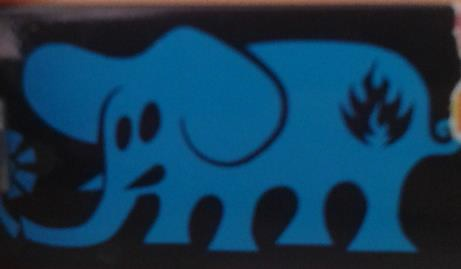## Tracking object

### Input (tracked object)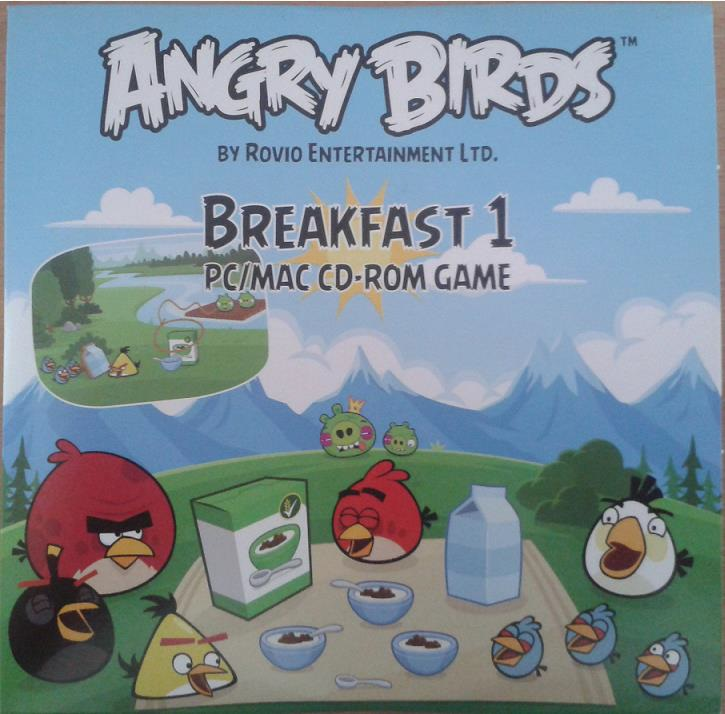## Modifying mask for deleting object

### Input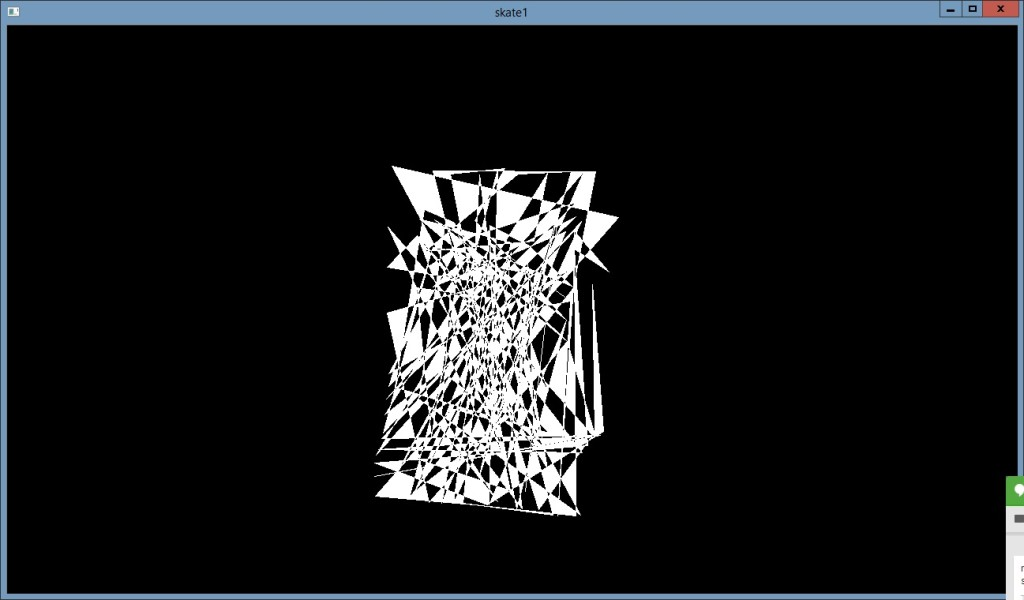### Output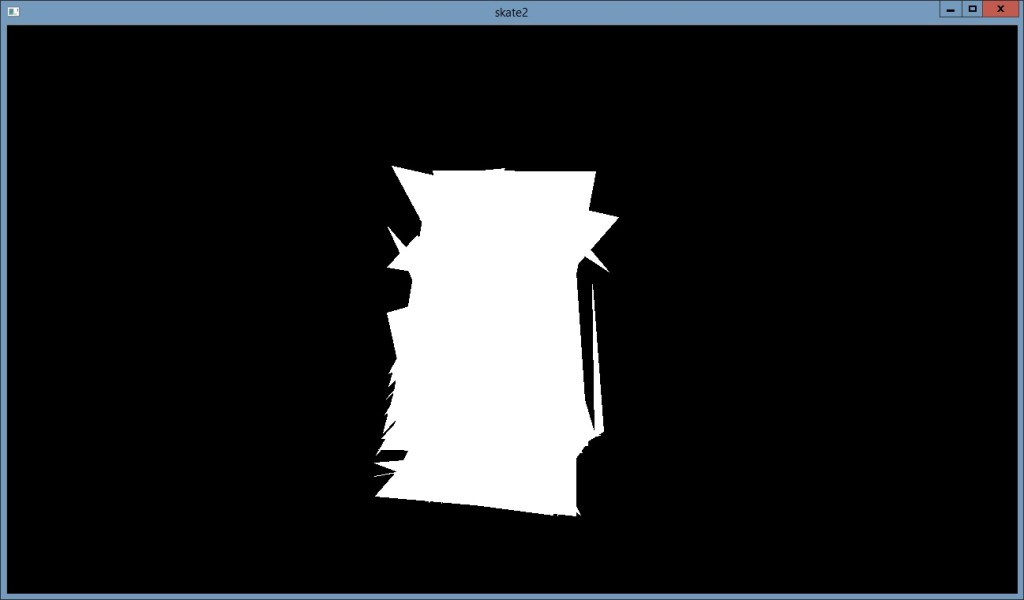## Deleting object

### Input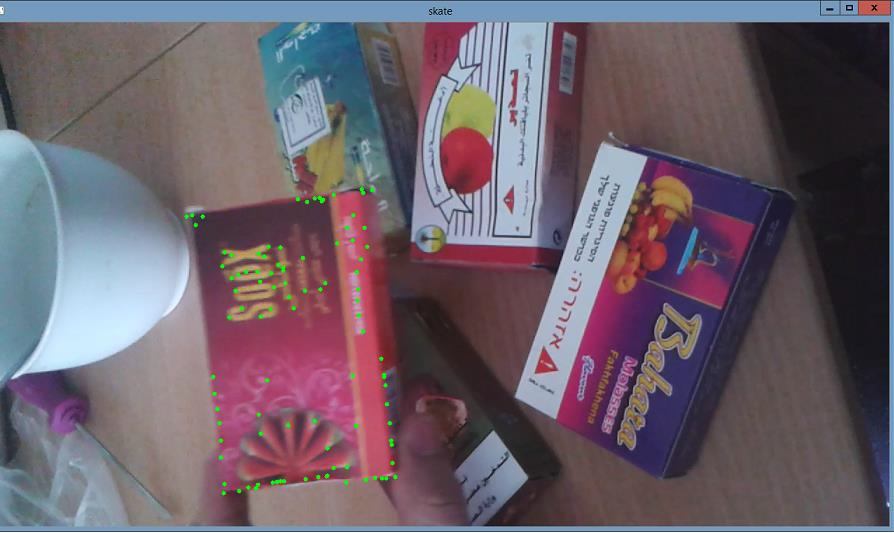### Output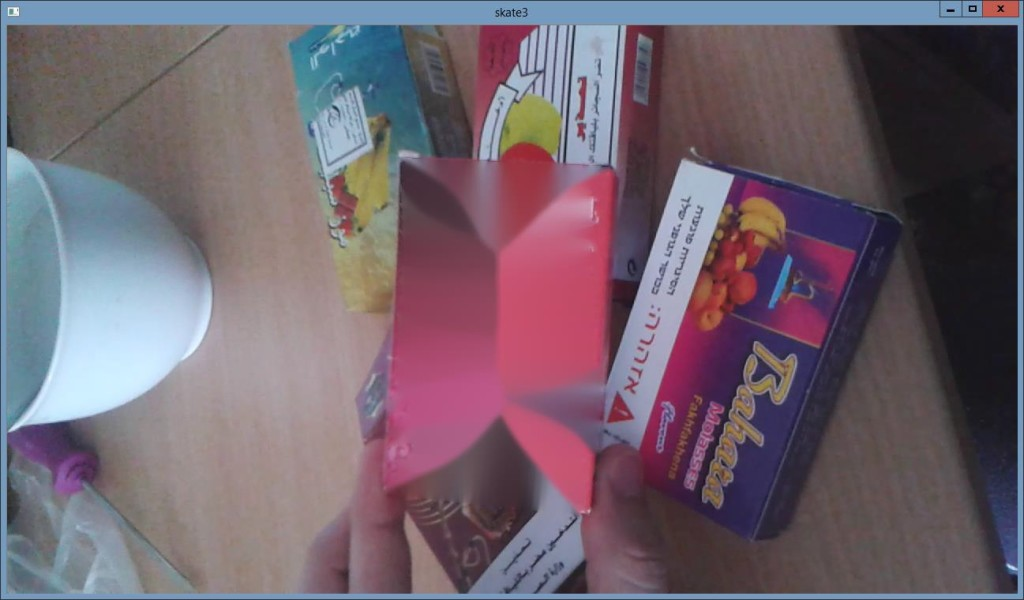Posted on

## Photo mergingMichal Lohnicky

This example shows how to merge two photos using OpenCV. SURF features are used to find a homography to align the images and histogram matching with Bhattacharyya distance is used for merging them seamlessly.

Functions used: cv.CalcHist, cv.FindHomography, cv.CompareHist(…, CV_COMP_BHATTACHARYYA), cv.ExtractSURF

## The process

1. Preprocessing
2. Image registration
3. Finding the correspondences between detected points
4. Calculating the homography
5. Histogram matching
6. Creating the blurred stitching mask

The matching process is demonstrated on the following images:

## Results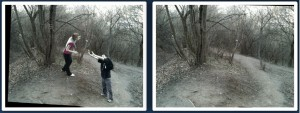Python source code is provided

Posted on## Description

The goal of this project is to create a program that will be able to stitch a sequence of images fromgoogle street-view and make movie from it. The idea came to my mind, when I needed to check thecrossroads and traffic signals along the route I’ve never driven before. The method was tointerpolatefew more images between two consecutive views to simulate moving car. To do that Ihad to do following steps:

## Process

1. Remove UI elements from images
2. Find homography between following images
3. Interpolate homography between them
4. Put images into movie

## Removing UI elements from images

Removing UI elements is important because in later steps I will need to find similar areas and those elements can spoil the match-up. First I cycled through all images and gained areas with same color in black. The resulting image was accumulated from all the differences. Black areas represent pixels that were same in all images. To improve the mask I inverted the image did some thresholding, Gaussian blur and again thresholding. Result was mask used for inpaint method to fill in those regions without UI elements with color.

## Example of process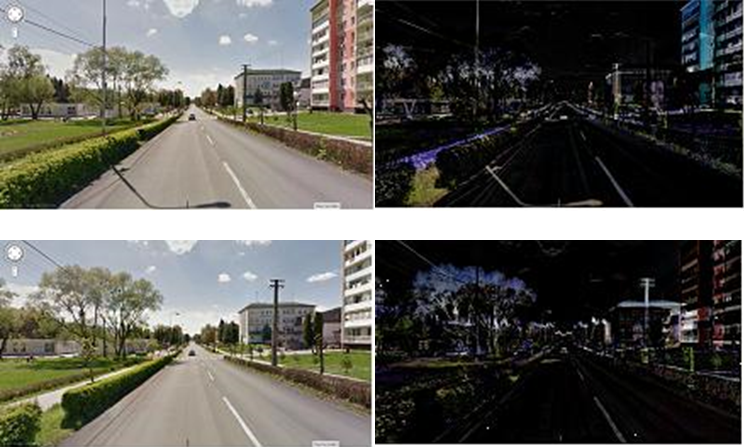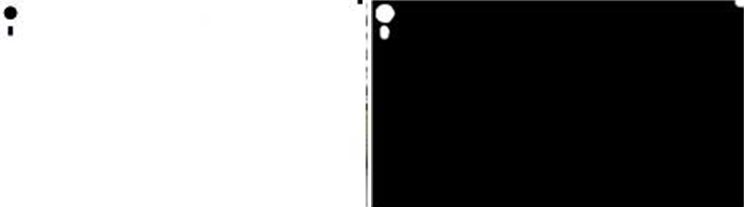## Finding homography

Homography found between two images was found using SURF detector. I improved the detection by using mask of similar areas as in previous step. I did it because many key-points were detected on sky or far objects and results were generally worse. Last step was to interpolate from one image to another using homography. In my program I used 25 steps between two pictures. Those pictures were stacked into movie and saved.

```Mat homo = findMatch(pic1, pic2);
bj_corners = cvPoint(0,0)
////-- Get the corners from the image_1 ( the object to be "detected" )
vector<Point2f> obj_corners(4);
obj_corners = cvPoint(0,0);
obj_corners = cvPoint( pic2.cols, 0 );
obj_corners = cvPoint( pic2.cols, pic2.rows );
obj_corners = cvPoint( 0, pic2.rows );

vector<Point2f> corners(4);
vector<Point2f> inter_corners(4);
perspectiveTransform( obj_corners, corners, homo);
...
...
for(int i=0; i<4; i++) {
inter_corners[i].x = corners[i].x - distance[i].x*j;
inter_corners[i].y = corners[i].y - distance[i].y*j;
}
Mat interHomo = findHomography( corners, inter_corners, 0 );
Mat transformed;
warpPerspective(pic1, transformed, interHomo, Size(600,350));
result[j] = transformed;
```
Posted on

## Detection and removal of circular artifacts from photographs## Description

Reflecting flashlight from dust, snowflakes or raindrops can produce irritating circular artifacts. For its detecting and removing we propose process, where we use improved circle detection besides using houghCircles function. For removing detected artifacts we use morphological reduction.

## Functions used

adaptiveThreshold, Canny, HoughCircles, findContours, fitEllipse, ImReconstruct

## Process

Limitation: Minimal circle size (15px )- Maximal circle size(30px)

```medianBlur()
OutputImg := InputImg + FilteredImg
```
2. Detection with HoughCircles
```Canny()
GaussianBlur()
HoughCircles()
Accept/ignore circles (based on size)
```
3. Detection with Morphological reconstruction and contour analysis
```mask := InputImg
marker := InputImg â€“ degreeOfMorphreduct
marker := inv(marker)
differenceImg := marker2 â€“ marker1
differenceImg := medianBlur(differenceImg)
differenceImg := threshold(differenceImg)
contour[] = findContours (differenceImg)
ellipse[i] := fitEllipse(contour[i])
accept/ignore circles (based on size and ellipse axes)
draw white ellipse[i]
draw black contour[i]
crop Regions Of Interest
opening(regionOfInterest[i])
if countNonZero(regionOfInterest[i]) > threshold then accept; else ignore;
```
4. Result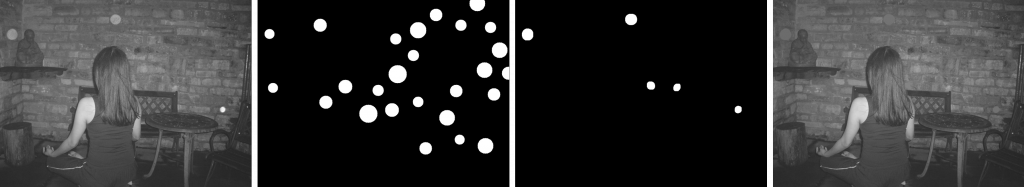Posted on

## Frequency domain filtrationThis post provides an example of image filtration and editing in the frequency domain. Practical methods of image spectrum conversion and Gaussian mask creation are described. The sample application is written in C++ using the OpenCV 2.x API. The full source code is also attached.

## Image data transformation into the frequency domain

In frequency domain we can analyze the spectrum of signals. Â The known Fourier transform is a linear transformation used for the conversion from time (e.g. audio signals in 1 dimension) or spatial (e.g. image in 2 dimensions) domain into the frequency domain. By the definition of Furier trasform is given, that the spectral Â data are complex numbers in general.OpenCV library include functions for calculation of Discrete Fourier Transform of an input image into a complex matrix – spectrum.
The next sample function shows a possibility to use the filtration in frequency domain for the enhancement of the image. Optimal DFT size was achieved by the method “zero padding”.
Note: Resolution of complex output can be increased by multiplying M and N variables with a constant.

```Mat computeDFT(Mat image) {
int m = getOptimalDFTSize(image.rows);
int n = getOptimalDFTSize(image.cols);
// create output image of optimal size
copyMakeBorder(image, padded, 0, m - image.rows, 0, n - image.cols, BORDER_CONSTANT, Scalar::all(0));
// copy the source image, on the border add zero values
// create a complex matrix
Mat complex;
merge(planes, 2, complex);
dft(complex, complex, DFT_COMPLEX_OUTPUT);Â  // fourier transform
return complex;
}
```

Once the complex matrix is generated, the visualization of spectrum can be done by Â the OpenCV operation Â magnitude (which is per-element matrix operation) and then by the conversion into the logarithmic scale for proper display.

Note: shift() function does the rearrangement of quadrants using SetROI() and CopyTo() functions of OpenCV API. See the full source code for more details.

```void updateMag(Mat complex) {
Mat magI;
Mat planes[] = {
Mat::zeros(complex.size(), CV_32F),
Mat::zeros(complex.size(), CV_32F)
};
split(complex, planes); // planes = Re(DFT(I)), planes = Im(DFT(I))
magnitude(planes, planes, magI); // sqrt(Re(DFT(I))^2 + Im(DFT(I))^2)
// switch to logarithmic scale: log(1 + magnitude)
magI += Scalar::all(1);
log(magI, magI);
// Transform the magnitude matrix into a viewable image (float values 0-1)
normalize(magI, magI, 1, 0, NORM_INF);
imshow("spectrum", magI);
}
```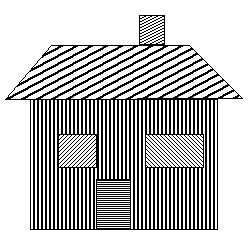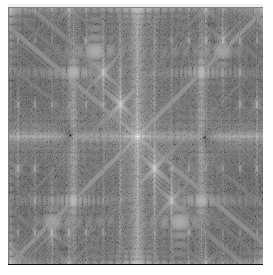## Processing

This project was designed as an interactive tool which can clear somelocal extremes in spectrum by multiplying it with aÂ GaussÂ filter. OpenCV framework provides functions to handle mouse clicks and input trackballs, please see the full source code for more details.

First of all we need to create a Gaussian mask, if we working with in frequency domain we need to consider that, the spectrum is symmetric, so the mask is also need to mirrored by image center.

For processing image in frequency domain, we would like use the Gaussian mask as a complex matrix and multiply it with the original imageâ€™s spectrum.

```Mat mask = createGausFilterMask(complex.size(), x, y, kernel_size, true, true);

Mat planes[] = {
Mat::zeros(complex.size(), CV_32F),
Mat::zeros(complex.size(), CV_32F)
};
Mat kernel_spec;
merge(planes, 2, kernel_spec);

mulSpectrums(complex, kernel_spec, complex, DFT_ROWS); //only DFT_ROWS flag is accepted
```

## Results

The backward transformation from the frequency domain is handled by using inverse DFT.

```void updateResult(Mat complex)
{
Mat result;
idft(complex, result);
// equivalent to:
// dft(complex, result, DFT_INVERSE + DFT_SCALE);
Mat planes[] = {
Mat::zeros(complex.size(), CV_32F),
Mat::zeros(complex.size(), CV_32F)
};
split(result, planes); // planes = Re(DFT(I)), planes = Im(DFT(I))
magnitude(planes, planes, result); // sqrt(Re(DFT(I))^2 + Im(DFT(I))^2)
normalize(result, result, 0, 1, NORM_MINMAX);
imshow("result", result);
}
```

## Practical samples

Watch the Sample screen video

Posted on

## Frequency domain filtration – source

```#include

using namespace cv;
using namespace std;

void onMouse( int event, int x, int y, int, void* param);
void updateMag(Mat complex);
void updateResult(Mat complex);

Mat computeDFT(Mat image);
Mat createGausFilterMask(Size mask_size, int x, int y, int ksize, bool normalization, bool invert);
void shift(Mat magI);

int kernel_size = 0;

int main( int argc, char** argv )
{

String file;
file = " << SAMPLE FILE >>";

namedWindow( "Orginal window", CV_WINDOW_AUTOSIZE  );// Create a window for display.
imshow( "Orginal window", image );                   // Show our image inside it.

Mat complex = computeDFT(image);

namedWindow( "spectrum", CV_WINDOW_AUTOSIZE );
createTrackbar( "Gausian kernel size", "spectrum", &kernel_size, 255, 0 );
setMouseCallback( "spectrum", onMouse, &complex);

updateMag(complex);			// compute magnitude of complex, switch to logarithmic scale and display...
updateResult(complex);		// do inverse transform and display the result image
waitKey(0);

return 0;
}

void onMouse( int event, int x, int y, int, void* param)
{
if( event != CV_EVENT_LBUTTONDOWN )
return;
// cast *param to use it local
Mat* p_complex = (Mat*) param;
Mat complex = *p_complex;

// show the kernel

Mat planes[] = {Mat::zeros(complex.size(), CV_32F), Mat::zeros(complex.size(), CV_32F)};
Mat kernel_spec;
merge(planes, 2, kernel_spec);

mulSpectrums(complex, kernel_spec, complex, DFT_ROWS); // only DFT_ROWS accepted

updateMag(complex);		// show spectrum
updateResult(complex);		// do inverse transform

*p_complex = complex;

return;
}

void updateResult(Mat complex)
{
Mat work;
idft(complex, work);
//	dft(complex, work, DFT_INVERSE + DFT_SCALE);
Mat planes[] = {Mat::zeros(complex.size(), CV_32F), Mat::zeros(complex.size(), CV_32F)};
split(work, planes);                // planes = Re(DFT(I)), planes = Im(DFT(I))

magnitude(planes, planes, work);	  // === sqrt(Re(DFT(I))^2 + Im(DFT(I))^2)
normalize(work, work, 0, 1, NORM_MINMAX);
imshow("result", work);
}

void updateMag(Mat complex )
{

Mat magI;
Mat planes[] = {Mat::zeros(complex.size(), CV_32F), Mat::zeros(complex.size(), CV_32F)};
split(complex, planes);                // planes = Re(DFT(I)), planes = Im(DFT(I))

magnitude(planes, planes, magI);    // sqrt(Re(DFT(I))^2 + Im(DFT(I))^2)

// switch to logarithmic scale: log(1 + magnitude)
magI += Scalar::all(1);
log(magI, magI);

shift(magI);
normalize(magI, magI, 1, 0, NORM_INF); // Transform the matrix with float values into a
// viewable image form (float between values 0 and 1).
imshow("spectrum", magI);
}

#include "dft_routines.h";

Mat computeDFT(Mat image) {
// http://opencv.itseez.com/doc/tutorials/core/discrete_fourier_transform/discrete_fourier_transform.html
Mat padded;                            //expand input image to optimal size
int m = getOptimalDFTSize( image.rows );
int n = getOptimalDFTSize( image.cols ); // on the border add zero values
copyMakeBorder(image, padded, 0, m - image.rows, 0, n - image.cols, BORDER_CONSTANT, Scalar::all(0));
Mat complex;
merge(planes, 2, complex);         // Add to the expanded another plane with zeros
dft(complex, complex, DFT_COMPLEX_OUTPUT);  // furier transform
return complex;
}

Mat createGausFilterMask(Size mask_size, int x, int y, int ksize, bool normalization, bool invert) {
// Some corrections if out of bounds
if(x < (ksize / 2)) {
ksize = x * 2;
}
if(y < (ksize / 2)) {
ksize = y * 2;
}
if(mask_size.width - x < ksize / 2 ) {
ksize = (mask_size.width - x ) * 2;
}
if(mask_size.height - y < ksize / 2 ) {
ksize = (mask_size.height - y) * 2;
}

// call openCV gaussian kernel generator
double sigma = -1;
Mat kernelX = getGaussianKernel(ksize, sigma, CV_32F);
Mat kernelY = getGaussianKernel(ksize, sigma, CV_32F);
// create 2d gaus
Mat kernel = kernelX * kernelY.t();

// copy kernel to mask on x,y
Mat pos(mask, Rect(x - ksize / 2, y - ksize / 2, ksize, ksize));
kernel.copyTo(pos);

Mat posi(maski, Rect(( mask_size.width - x) - ksize / 2, (mask_size.height - y) - ksize / 2, ksize, ksize));
kernel.copyTo(posi);

// transform mask to range 0..1
if(normalization) {
}

if(invert) {
}

}

void shift(Mat magI) {

// crop if it has an odd number of rows or columns
magI = magI(Rect(0, 0, magI.cols & -2, magI.rows & -2));

int cx = magI.cols/2;
int cy = magI.rows/2;

Mat q0(magI, Rect(0, 0, cx, cy));   // Top-Left - Create a ROI per quadrant
Mat q1(magI, Rect(cx, 0, cx, cy));  // Top-Right
Mat q2(magI, Rect(0, cy, cx, cy));  // Bottom-Left
Mat q3(magI, Rect(cx, cy, cx, cy)); // Bottom-Right

Mat tmp;                            // swap quadrants (Top-Left with Bottom-Right)
q0.copyTo(tmp);
q3.copyTo(q0);
tmp.copyTo(q3);
q1.copyTo(tmp);                     // swap quadrant (Top-Right with Bottom-Left)
q2.copyTo(q1);
tmp.copyTo(q2);
}```